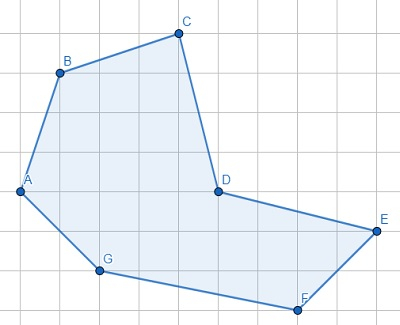# Program to check points are forming concave polygon or not in Python

Suppose we have outer points of a polygon in clockwise order. We have to check these points are forming a convex polygon or not. A polygon is said to be concave if any one of its interior angle is greater than 180°.From this diagram it is clear that for each three consecutive points the interior angle is not more than 180° except CDE.

So, if the input is like points = [(3,4), (4,7),(7,8),(8,4),(12,3),(10,1),(5,2)], then the output will be True.

To solve this, we will follow these steps −

• n := size of points
• for i in range 0 to size of points, do
• p1 := points[i-2] when i > 1, otherwise points[n-2]
• p2 := points[i-2] when i > 0, otherwise points[n-1]
• p3 := points[i]
• if angle between points (p1, p2, p3) > 180, then
• return True
• return False

## Example

Let us see the following implementation to get better understanding −

import math
def get_angle(a, b, c):
angle = math.degrees(math.atan2(c-b, c-b) - math.atan2(a-b, a-b))
return angle + 360 if angle < 0 else angle

def solve(points):
n = len(points)
for i in range(len(points)):
p1 = points[i-2]
p2 = points[i-1]
p3 = points[i]
if get_angle(p1, p2, p3) > 180:
return True
return False

points = [(3,4), (4,7),(7,8),(8,4),(12,3),(10,1),(5,2)]
print(solve(points))

## Input

[(3,4), (4,7),(7,8),(8,4),(12,3),(10,1),(5,2)]


## Output

True